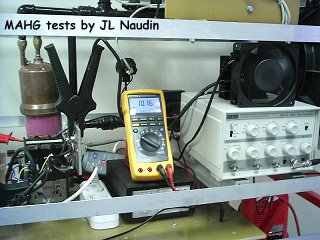Free Energy from Atomic Hydrogen
MAHG - Towards High Efficiency
Created on June 20, 2005 - Updated on June 23, 2005

The purpose of these tests is to improve the MAHG efficiency so as to overcome the losses due to the poor efficiency of the Carnot cycle at the MAHG output.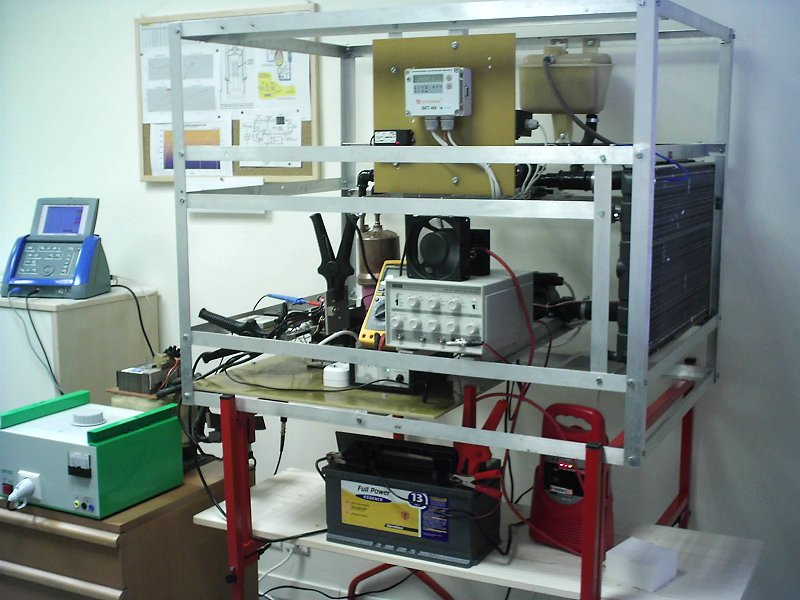The MAHG under the High Efficiency tests runs with short DC pulses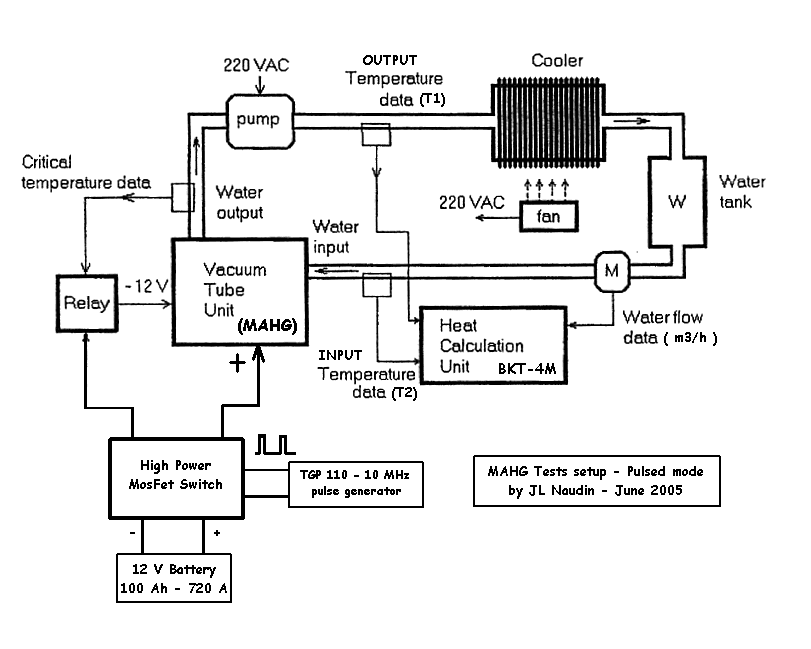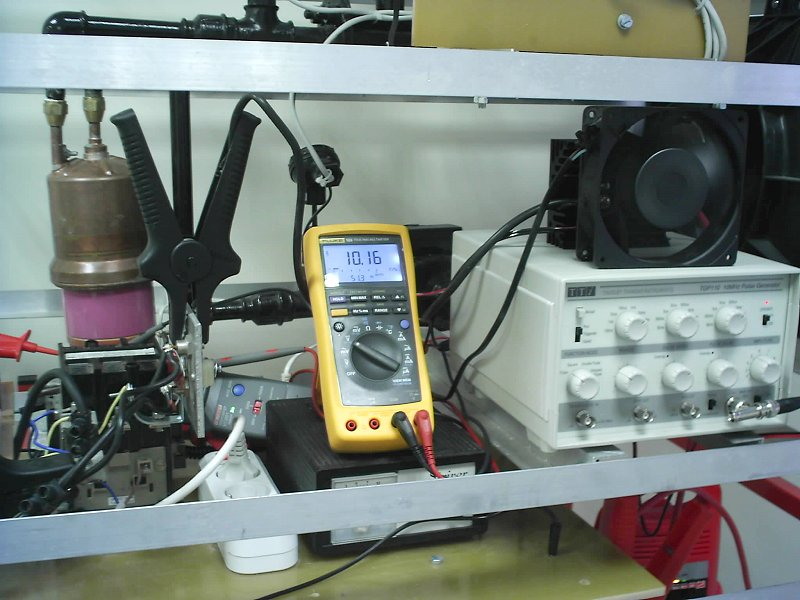The previous power supply has been replaced by a high power battery (12V 100Ah - 720A) connected through a High Power MosFet Switcher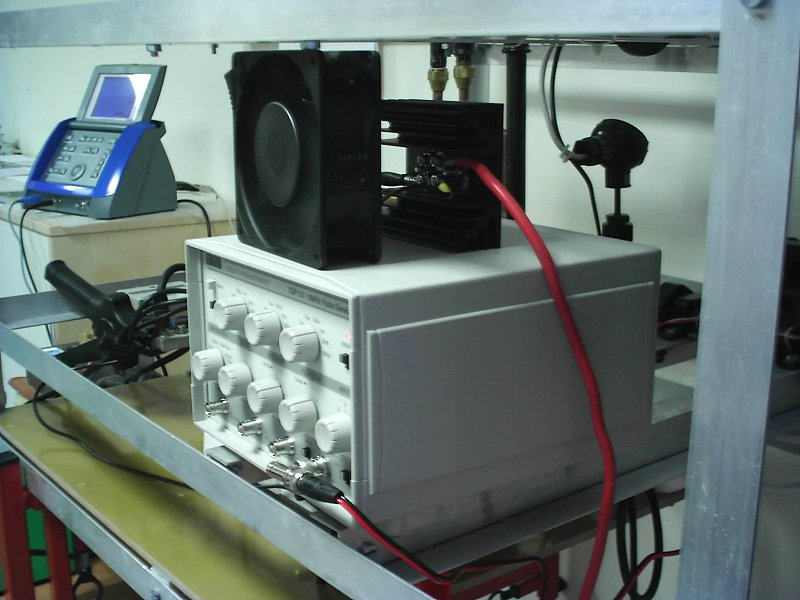The High Power Switcher is driven with a TGP 110 - 10 MHz pulse generator (see below)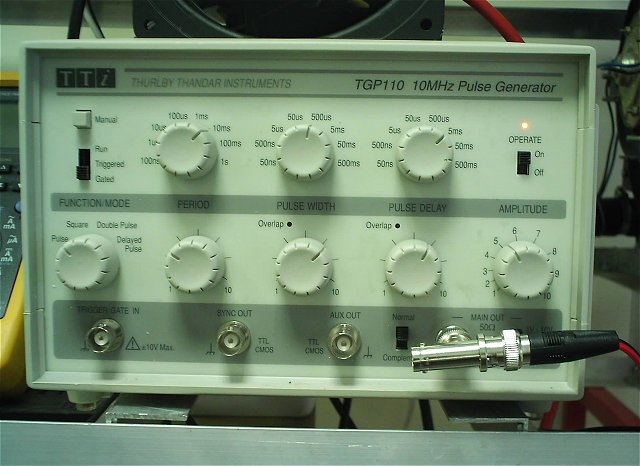Test procedure :

• 1) Before each RUN, the MAHG device is cooled with the fan for at least 30 min so as to get a stable Input/Output temperature.

• 2) The initial conditions are carefully noted : the initial water flow (S_F_m3/h), the inital temperature (Ts2) at the input and at the output (Ts1) and the temperature correction value (T Offset = Ts1 - Ts2) for the initial conditions is calculated.

• 3) At the end of the long RUN the final conditions are noted : the final water flow (F_F_m3/h), the final temperature (Tf2) at the input and at the output (Tf1) and the temperature difference (dT = (Tf1 - Tf2) - (T Offset)) is calculated. The average voltage and current are also measured on the digital oscilloscope.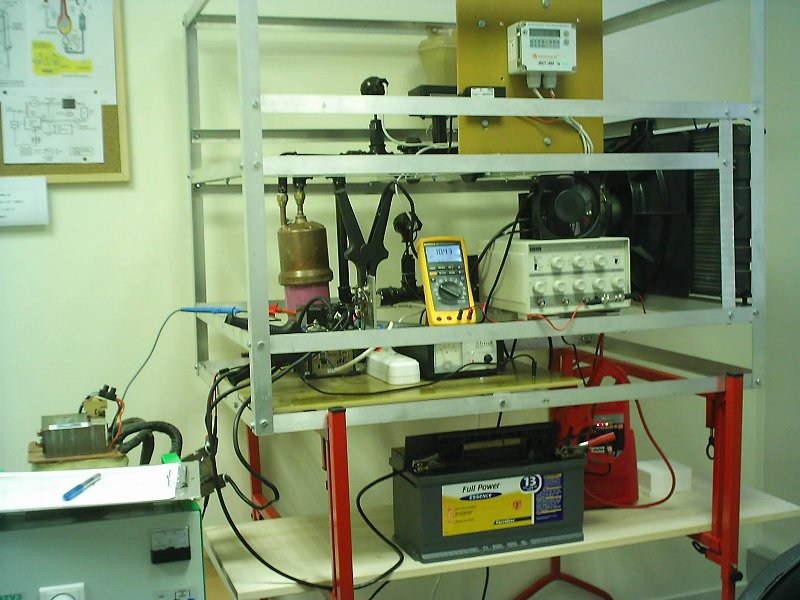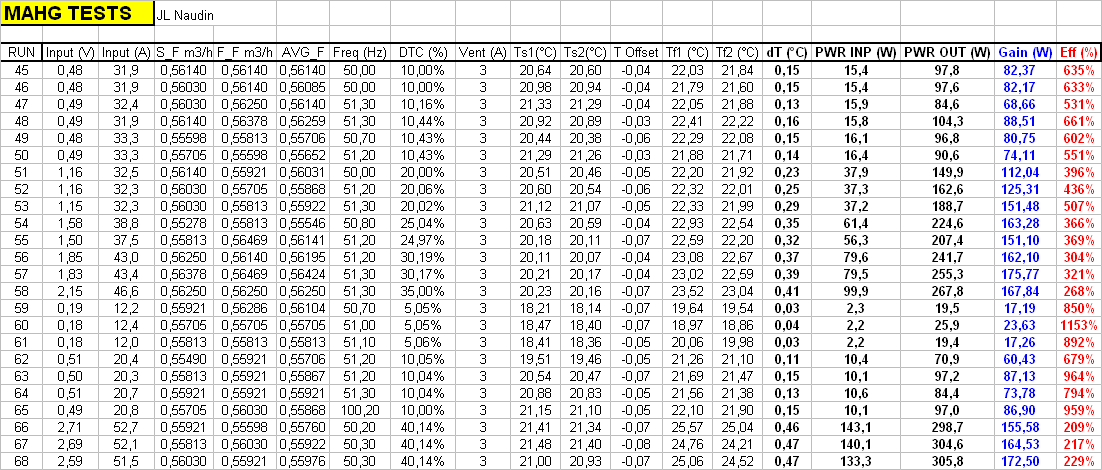Data sheet results details :

• Input (V), Input (A) : Rms Voltage and Rms Current at the input

• S_F_m3/h : Water flow measured at the start of the run in m3/h

• F_F_m3/h : Water flow measured at the end of the run in m3/h

• Freq : Pulse frequency ( square wave )

• DTC : Duty Cycle of the pulse

• AVG_F : Average flow of water during all the run in m3/h

• Ts1 : Output temperature of the water at the start of the run

• Ts2 : Input temperature of the water at the start of the run

• T Offset = Ts1 - Ts2 : temperature correction value for the initial conditions

• Tf1 : Output temperature of the water at the end of the run

• Tf2 : Input temperature of the water at the end of the run

• dT : Temperature difference at the end of the run, dT = (Tf1 - Tf2) - (T Offset)

• PWR INP : Electrical power at the input

• PWR OUT : Thermal power at the output :

• Power OUTPUT = Flow Rate (m3/h) x dT (°C ) x ( 4,18 x 106 / 3600 ) = Flow Rate (m3/h) x dT (°C ) x 1161,11

Efficiency = Power OUTPUT / Power INPUT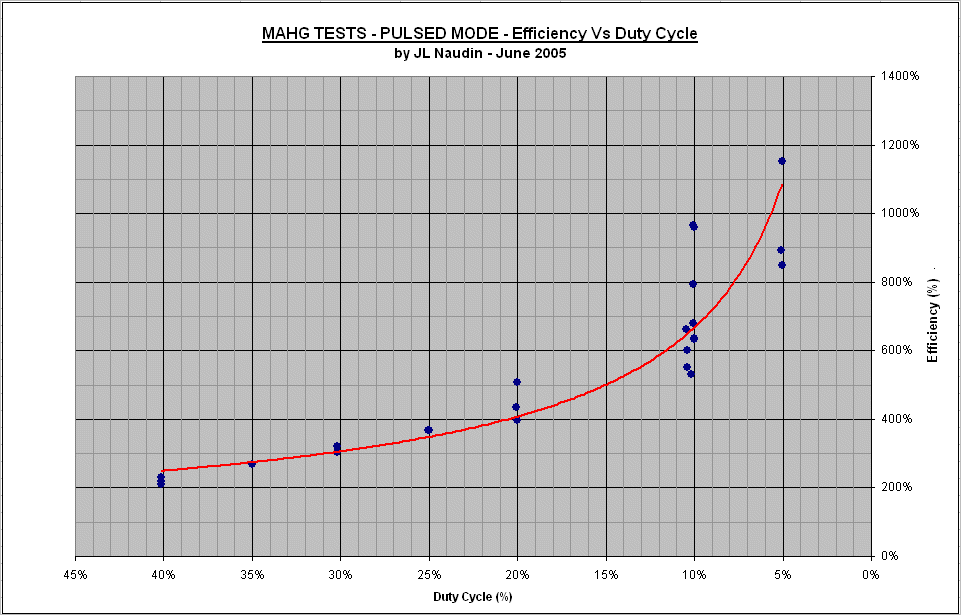Comments from Jean-Louis Naudin : These tests demonstrate clearly that the efficiency of the MAHG increases ( up to 1153% ) while the duty cycle of the squared pulse decreases (up to 5%).

LONG TESTS RUNS ( 2 hours ) in PULSED MODE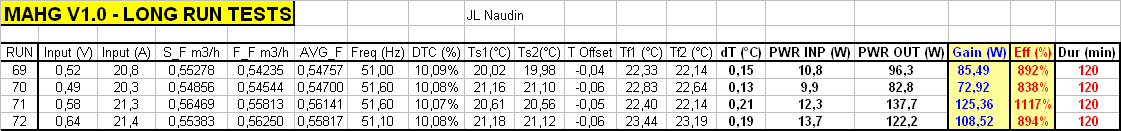Comments from Jean-Louis Naudin : Long tests runs ( 2 hours ) have been performed at 10% DTC. These tests have demonstrated that the efficiency is greater than the previous 10 min tests runs. This was due to the warm up time of the MAHG at low power ( ~10 Watts input ), so after 2 hours the power output was stable. In a next version of the MAHG setup (v2.0), I shall use a new digital flowmeter and a new thermologger with two brand new thermocouples (type K). The water flow will be also decreased so as to increase the dT at the MAHG output. All the data will be recorded in real time for a better accuracy of the measurements.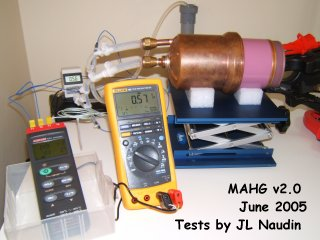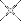HIGH EFFICIENCY confirmed with a new tests bench